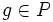# Extraspecial implies Camina

(diff) ← Older revision | Latest revision (diff) | Newer revision → (diff)
Jump to: navigation, search
This article gives the statement and possibly, proof, of an implication relation between two group properties. That is, it states that every group satisfying the first group property (i.e., extraspecial group) must also satisfy the second group property (i.e., Camina group)
View all group property implications | View all group property non-implications
Get more facts about extraspecial group|Get more facts about Camina group

## Statement

Any extraspecial group is a Camina group. In other words, if$P$ is a group of prime power order (or more generally, a possibly infinite p-group) such that the center, Frattini subgroup, and commutator subgroup of$P$ all coincide and are cyclic of prime order, then$P$ is a Camina group.

## Proof

Given: A$p$-group$P$ such that$Z(P) = \Phi(P) = [P,P]$ is cyclic of prime order.

To prove: Every coset of$[P,P]$ other than$[P,P]$ itself is a conjugacy class.

Proof: Since$[P,P]$ is of order$p$, so is every coset of it. Each coset of$[P,P]$ is a union of conjugacy classes (since nay two conjugate elements are in the same coset of$[P,P]$. Thus, the size of each conjugacy class is at most$p$. On the other hand, the conjugacy class of$g \in P$ is in bijection with the coset space of$C_P(g)$. Since$P$ is a$p$-group, any subgroup of finite index has index a power of$p$, so the size of every conjugacy class is a power of$p$. Thus, the only possible sizes of conjugacy classes are$1$ and$p$.

However, since$Z(P) = [P,P]$, no element outside$[P,P]$ has a conjugacy class of size one, forcing all conjugacy classes outside to have size$p$. Hence, for any element outside$[P,P]$, its conjugacy class equals its$[P,P]$-coset.